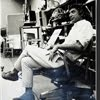## Eugene Ray SDSU 1970'sEugene Ray 1970's. photo credit: Tom Davis

## Wednesday, January 14, 2015

### ELECTRO VIBRATORY BODY / john dee, alchemist / "mr turner"

+COLOR LIGHT ENERGY+
>painting with radiant energy is an approach<
>to constructing environment that has been<
+SYNERGETIC ENVIRON)+
>a fascination for me for many long years<
^^^^^^^^^^^^^^^^^^^^^^^^^^^
+BIOTRONIC ENERGY+
>as you know somehow became the focus<
>of a young scholar from hong kong, wun<
+"THE GOD'S MACHINES")+
>chok bong who produced an amazing<
>book that >corroborated my research<
^^^^^^^^^^^^^^^^^^^^^^^

>was my title for an approach to designing<
>buildings that utilized electromagnetic<
+>/ BIOTRONIC ENERGY)+
>biotronic energy in several foremats<
^^^^^^^^^^^^^^^^^^^^^^^>the brain is an amazing mechanism and the<
>"third eye" >penial gland< is a fantastic<
+>>> / PENIAL GLAND)+
>instrument of very magical proportions<
>and perhaps the source of et influence<
^^^^^^^^^^^^^^^^^^^^^^
>this flower bud photographed w/ kerlian<
>techniques would reveal the force field<
+>> / BIO FORCE FIELD)+
>of biotronic energy surrounding the tip<
^^^^^^^^^^^^^^^^^^^^^^^
>john dee (above) & sir isaac newton are >two<
>of the most brilliant english scientist-alchemist<
>magician's to be found in recorded history &<
>dee was also a >mathematician & astrologer<
^^^^^^^^^^^^^^^^^^^^^^^^^^^
>you can imagine the astonishment & gratitude<
>i felt to wun chok bong for dedicating his two,
>volume recent research into medieval church,
^^^^^^^^^^^^^^^^^^^^^^^^^^^
>the fascinating factor for me is that a vision<
>for radiant architecture can be traced to the<
+(WUN CHOK BONG KNEW+
+MEDIEVAL RESOURCES)+
>work of our ancestors in the orient forest /<
>bernard de clairvaux sacred architecture<
^^^^^^^^^^^^^^^^^^^^^^^^
>my concept for a residence designed as a<
>holistic synergetic environment w/ strong<
+/ UNIVERSAL SPHEROID)+
>organic healing force fields would please<
>john dee & isaac newton >if alive today<
^^^^^^^^^^^^^^^^^^^^^^^^^
>the open minds >international ufo congress<
>february 18 to 21 at fort mcdowell arizona<
+ / BACK ENGINEERING)+
>could benefit from a presentation on the<
>many et back engineering contributions<
^^^^^^^^^^^^^^^^^^^^^^^^
>as wun chok bong has shown in his seminal<
>book "the god's machines"the >djed energy<
+(ERIC VON DANIKEN W/+
+DJED ENERGY DEVICE)+
>device was located as a key energy source<
>in important ancient egyptian monuments<
^^^^^^^^^^^^^^^^^^^^^^^^^^^>brilliant english master machinist, and energy<
>researcher christopher dunn has illustrated in<
+>> / PYRAMID POWER)+
>his books how the egyptian pyramids were<
>huge energy sources verifying bong research<
^^^^^^^^^^^^^^^^^^^^^^^^^^
>"your electro vibratory body" by victor beasley<
>& christopher hill was just one of many books<
+>>>>VIBRATORY BODY)+
>about >health & electro-magnetic energy that<
>was important to my 70s research / teaching<
^^^^^^^^^^^^^^^^^^^^^^^^

>google has done an amazing job of collecting<
>the blog images relating to my radiant design<
>prototypes based on >electromagnetic energy<
>force fields resulting in >radiant architecture<
^^^^^^^^^^^^^^^^^^^^^^^^^^^

+"MR TURNER"
>the fine current film about the great english<
>artist j.m.w. turner portrays how a >vision<
>of a very new, almost abstract kind can<
>progender what is now >"modern art"<
^^^^^^^^^^^^^^^^^^^^^
+COSMIC CONSCIOUSNESS+
+WAS HIS >GALVENIZING+
>(organic biotronic<
+FUEL IN LIFE ON EARTH+ !
^^^^^^^^^^^^^^^^^^^
eugene ray, mfa, architect
professor emeritus, sdsu